# JEE Main Centre of Mass Previous Year Questions with Solutions

JEE Main previous year questions with solutions on topic centre of mass are available here. Practising JEE Main Previous Year Papers Questions of Physics will help all the JEE aspirants in understanding the question pattern as well as help in analyzing their weak & strong areas.

The point at which the entire mass of the body or the system of a particle is concentrated is called the centre of the mass of a body or system of a particle. The motion of the centre of mass characterizes the motion of the entire object. The centre of mass may or may not be the same as the geometric centre if a rigid body is considered. It is considered as a reference point for many other calculations of mechanics. The centre of mass of a rigid body is a point whose position is fixed with respect to the body as a whole. The concept of centre of mass (COM) is useful in analyzing the complicated motion of the system of objects, particularly when two and more objects collide or an object explodes into fragments.

## JEE Main Previous Year Solved Questions on Centre of Mass

Q1: Two blocks of masses 10 kg and 4 kg are con­nected by a spring of negligible mass and placed on a frictionless horizontal surface. An impulse gives a velocity of 14 m/s to the heavier block in the direction of the lighter block. The velocity of the centre of mass is

(a) 30 m/s

(b) 20 m/s

(c) 10 m/s

(d) 5 m/s

Solution:

Just after collision

vc= m1v1 + m2v2/m1 + m2

vc= (10 x 14 + 4 x 0)/(10+4)

vc= 10 m/s

Q2: A 20g bullet pierces through a plate of mass M1=1kg and then comes to rest inside the second plate of mass M2 = 2.98 kg, as shown in the figure. It is found that the two plates initially at rest, now move with equal velocities. Find the percentage loss in the initial velocity of the bullet when it is between M1 and M2. Neglect any loss of material of the plates due to the action of a bullet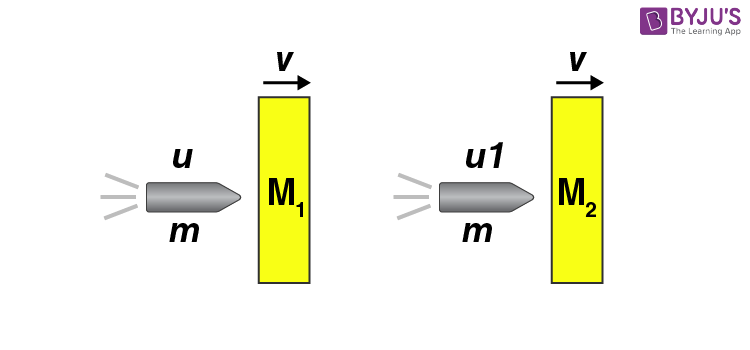(a) 50%

(b) 25%

(c) 100%

(d) 75%

Solution:

Let the initial velocity of the bullet = V1 m/s

The velocity with which each plate moves = V2 m/s

Applying conservation of momentum, the initial momentum of the bullet is equal to the sum of the final momentum of the plate M1 and the momentum of the second plate including the bullet.

after piercing M

∴ mV1 = M1V2 + (M2 + m)V2

0.02V1 = 1 x V2 + (2.98 + 0.02)V2

0.02V1 = 1 x V2 + 3V2

0.02V1 = 1 x V2 + 3V2

V1 = 4 V2 /0.02

V1 = 200V2 ———-(1)

Let the velocity of the bullet when it comes out of the first plate = V3

The momentum of the bullet on the first and the second plate is equal to the sum of the momentum of the second plate and the bullet.

0.02V3 = (0.02 + 2.980 V2) = 0.02 V3 = 3V2

V3= 150V2———-(2)

Loss percentage in the initial velocity of the bullet when it is moving between m1 and m2 is expressed as the following

Loss % ={(V1 – V3)/V1 } x 100

Loss % = {(200V2 – 150V2 )/200V2 } x 100

Loss % = {(200 – 150 )V2/200V2} x 100

Loss % = {(50 )/200} x 100

Loss % = 25%

Q3: Two particles A and B, initially at rest, move to­wards each other under the mutual force of attraction. At the instant when the speed of A is v and the speed of B is 2v, the speed of the centre of mass of the system is

(a) 3v

(b) v

(c) 1.5v

(d) zero

Solution

FA is the force on particle A

FA = mAaA = mAv/t

FB is the force on particle B

FB = mBaB = mB2v/t

Since FA= FB

mAv/t = mB2v/t

So mA = 2mB

For the centre of mass of the system

v = (mAvA + mBvB)/(mA + mB)

v = (2mAv – mB2v)/(2mB + mB) = 0

The negative sign is used because the particles are travelling in the opposite directions

Q4: Two small particles of equal masses start moving in opposite directions from a point A in a horizontal circular orbit. Their tangential velocities are v and 2v, respectively, as shown in the figure. Between collisions, the particles move with constant speeds. After making how many elastic collisions, other than that at A, will these two particles again reach the point A?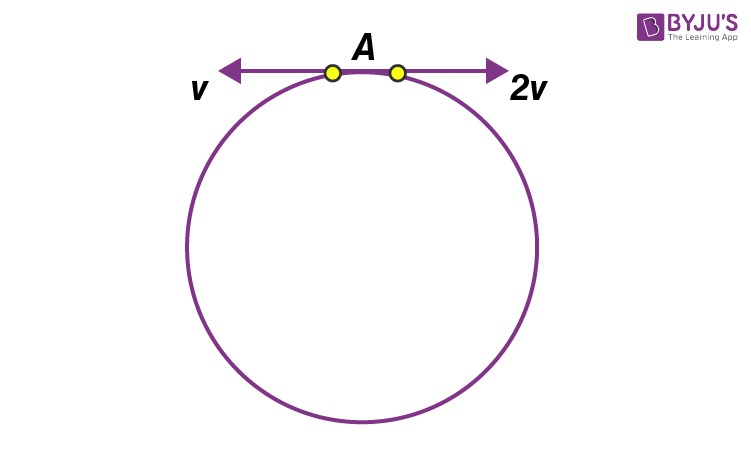(a) 4

(b) 3

(c) 2

(d) 1

Solution:

Let L be the circumference of the circle

After both the particles have left from A, the particle on the left will have a velocity v and the particle on the right will have a velocity 2v.

They will first meet at point B travelling L and 2L distance.

After collision velocity will get interchanged, the body with velocity will travel with a velocity 2v now and the one having velocity 2v will travel with velocity v.

So the next collision will happen at point D travelling 2L distance and L distance.

Again the velocity will get interchanged and they will collide at point A again.

So total it will be 2 collisions before they collide again at point A.

Q5: A point mass of 1 kg collides elastically with a stationary point mass of 5 kg. After their collision, the 1 kg mass reverses its direction and moves with a speed of 2 ms4. Which of the following statements(s) are correct for the system of these two masses?

1. The total momentum of the system is 3 kg ms-1
2. The momentum of 5kg mass after the collision is 4kg ms-1
3. The kinetic energy of the centre of mass is 0.75 J
4. The total kinetic energy of the system is 4 J

Solution: If the velocity of 1 kg mass before the collision is u and velocity of 5 kg mass after the collision is v then

From the conservation of linear momentum

1 x u + 5 x 0 = 1 x (-2) + 5 x v

u = -2 + 5v

Since the collision is elastic, total kinetic energy will be equal

Therefore,

½ x 1 x u2 = ½ x 1 x 22 + ½ x 5 x v2

u2 = 4 + 5v2

(-2 + 5v)2 = 4 + 5v2

4 – 20v + 25v2 = 0

-20v + 20v2 =0

-20v (1-v) =0

v = 1m/s

Thus, u=3m/s

The velocity of the centres of mass of the combined system

Vcm = ( m1v1+ m2v2)/(m1+m2)

Vcm = (1 x (-2)+5 x 1)/(1+5)

Vcm = 3/6

Vcm

The combined Kinetic Energy of the system

Kinetic Energy= ½ (1+5) x (½)2

K.E = 6/8

Kinetic Energy = 0.75 J

Total momentum of the system

= (m1 +m2)vcm

=(1+ 5) x ½

= 3 kg-m/s

Q6: A circular plate of uniform thickness has a diameter of 56cm. A circular portion of diameter 42cm is removed from one edge of the plate as shown in the figure. Find the position of the centre of mass of the remaining portion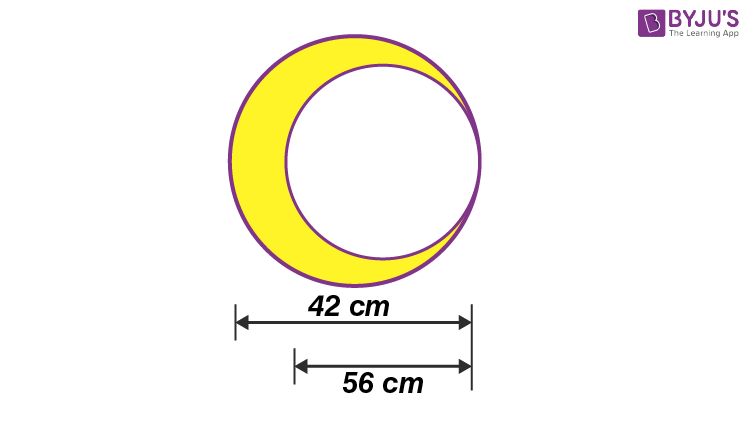Solution:

M is the mass of the original disc

M1 is the mass of the removed disc

If mass per unit area is m then,

Mass of original disc M = m x π x (28 x 10-2)2

Mass of removed disc M1 = m x π x (21 x 10-2)2

Mass of the remaining part = M – M1

centre of mass position of the original disc = 0 (at origin)

centre of mass position of the removed disc r1= 28 – 21 = 7cm

centre of mass of the remaining part= r2

Therefore,

M x 0 = M1r1 + (M – M1)r2

M1r1 = – (M – M1)r2

r2= (M1r1)/- (M – M1)

r2= [{(m π (21 x 10-2)2}/{-m π(28 x 10-2)2-m π (21 x 10-2)2 }]x7

r2= [(21)2 / (-343)]x7

r2= – 9 cm

Answer: 9 cm to the left of the bigger circle

Q7: Consider a rubber ball freely falling from a height h = 4.9 m onto a horizontal elastic plate. Assume that the duration of a collision is negligible and the collision with the plate is totally elastic. Then the velocity as a function of time and the height as a function of time will be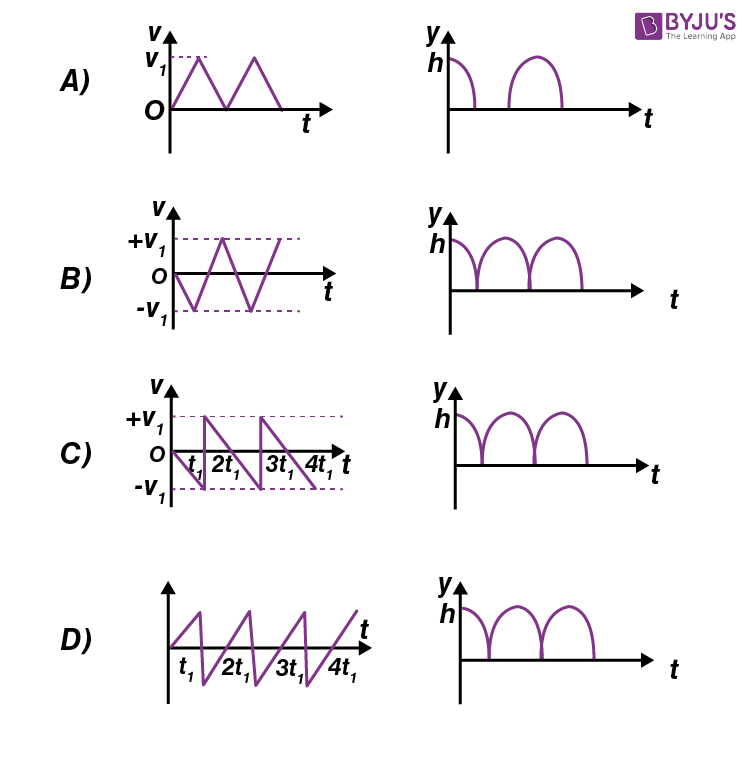Solution:

The velocity of the ball is reversed when it strikes the surface

Answer: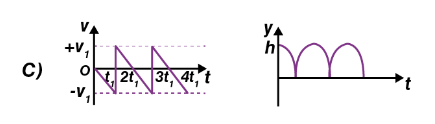Q8: Statement-1: Two particles moving in the same direction do not lose all their energy in a completely inelastic collision.

Statement-2: Principle of conservation of momentum holds true for all kinds of collisions.

(a) Statement–1 is true, Statement–2 is false

(b) Statement–1 is true, Statement–2 is true; Statement–2 is the correct explanation of Statement–1

(c) Statement–1 is true, Statement–2 is true; Statement–2 is not the correct explanation

of Statement–1

(d) Statement–1 is false, Statement–2 is true

Solution

In a completely inelastic collision then m1v1 + m2v2 = m1v + m2v

v = (m1v1 + m2v2)/(m1 + m2)

Kinetic Energy = p12/2m1 + p22/2m2

As p1 and p2 both cannot be zero simultaneously therefore total kinetic energy cannot be lost.

Answer: (b) Statement–1 is true, Statement–2 is true; Statement–2 is the correct explanation of Statement–1

Q9: A particle of mass m moving in the x-direction with speed 2v is hit by another particle of mass 2m moving in the y-direction with speed v. If the collisions are perfectly inelastic, the percentage loss in the energy during the collision is close to

(a) 56%

(b) 62%

(c) 44%

(d) 50%

Solution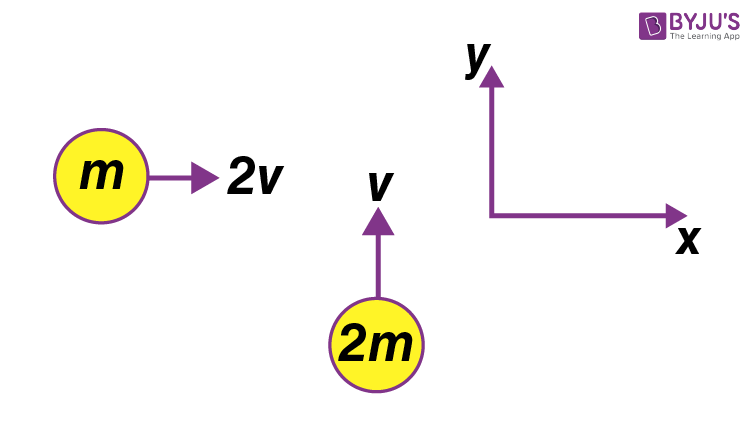Conservation of linear momentum in the x-direction

(pi)x = (pf)x or 2mv = (2m+m)Vx

vx = ⅔ v

Conservation of linear momentum in y-direction

(pi)y = (pf)y or 2mv = (2m+m)Vy

vy = ⅔ v

The initial kinetic energy of the two particles system is

Ei = ½ m(2v)2 + ½ (2m)v2

Ei = ½ x 4mv2 + ½ x 2mv2

Ei = 2mv2 +mv2 = 3mv2

Final energy of the combined two particles system is

Ef= ½ (3m)(vx2 + vy2)

Ef= ½ (3m)[4v2/9 + 4v2/9]

Ef= 4mv2/3

Loss of energy ΔE = Ei – Ef

ΔE = mv2(3 – 4/3)= (5/3)mv2

Percentage loss in the energy during the collision

ΔE/Ei x 100 = {(5/3)mv2/3mv2} x 100

=(5/9) x 100 ≃56%

Q10: The distance of the centre of mass of a solid uniform cone from its vertex is Z0. If the radius of its base is R and its height is h then Z0 is equal to

(a) 3h/4

(b) h2/4R

(c) 5h/8

(d) 3h2/8R

Solution

For a solid cone centre of mass from the base is h/4

Position of centre of mass of a solid cone from the vertex = h – h/4 = 3h/4

Q11: It is found that if a neutron suffers an elastic collinear collision with deuterium at rest, fractional loss of its energy is Pd; while for its similar collision with carbon nucleus at rest, fractional loss of energy is Pc. The values of Pd and Pc are respectively :

(a) (0.28,0 .89)

(b) (0, 0)

(c) (0, 1)

(d) (0.89,0.28)

Solution

Let the initial speed of neutron is v0 and kinetic energy is K

First collision: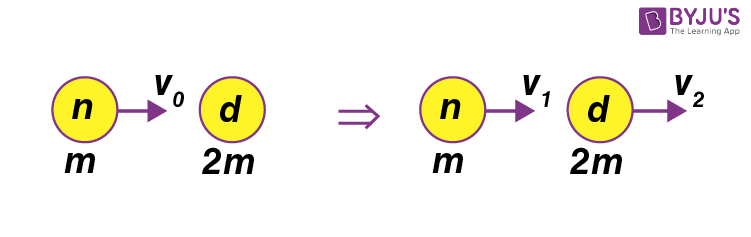By momentum conservation

mv0 = mv1 + 2mv2 ⇒ v0 = v1 + 2v2

By e = 1 v2 – v1 = v0

v2 = 2v0/3

v1= – v0/3

Frictional loss = {½ mv02 – ½ m(v0/3)2}/½ mv02

Pd = 8/9 ≈ 0.89

Second Collision: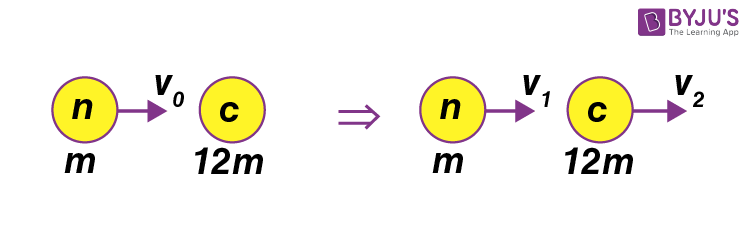By momentum conservation

mv0 = mv1 + 12mv2

v1 + 12v2 = v0

By e =1 v2 – v1 = v0

v2 = 2v0 / 13

v1 = -11v0/13

Now fraction loss of energy

Pc = [½ mv02 – ½ m(11v0/13)2] /1/2mv02

Pc = 48/169 = 0.28

Q12: In a collinear collision, a particle with an initial speed V0 strikes a stationary particle of the same mass. If the final total kinetic energy is 50% greater than the original kinetic energy, the magnitude of the relative velocity between the two particles, after the collision, is

(a) √2 v0

(b) v0/2

(c) v0/√2

(d) v0/4

Solution

Total kinetic energy after the collision = ½ mv12 + ½ mv22= 3/2(½ mv02)

v12 + v22= (3/2)v02 ——–(1)

By momentum conservation

mv0 = m(v1+v2) ——–(2)

(v1+v2)2 =v02

v12+v22+ 2v1v2= v02

2v1v2 = – v02/2

(v1 – v2)2 = v12+v22 -2v1v2=(3/2)v02 + v02/2

v1 – v2 = √2 v0

Q13: Two blocks A and B, each of mass m, are connected by a massless spring of natural length L and spring constant K. The blocks are initially resting on a smooth horizontal floor with the spring at its natural length, as shown in the figure. A third identical block C, also of mass m, moves on the floor with a speed v along the line joining A and B and collides elastically with A. Then

(a) The KE of the AB system at maximum compression of the spring is zero.

(b) The KE of the AB system at maximum compression of the spring is (¼ mv2)

(c) The maximum compression of the spring is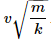(d) The maximum compression of the spring is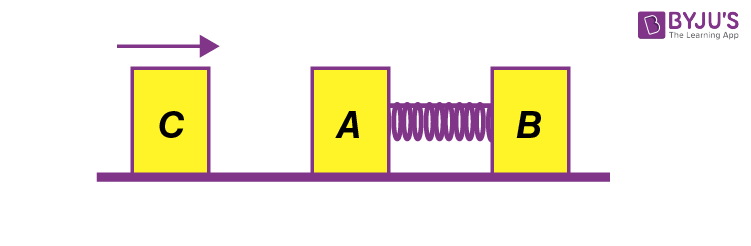Solution

There is an elastic collision between C and A

In an elastic collision, the velocities are exchanged if masses are the same.

∴ after the collision;

VC = 0 VA = v

Now the maximum compression will occur when both the masses A and B move with the same velocity.

∴ mv = (m + m) V (for system of A – B and spring)

∴ V = v/2

∴ KE of the A – B system = 1/2 x 2m (v/2)2 = mv2/4

And at the time of maximum compression.

½ mv2 = ½ x 2m(v/2)2 + ½ kx2max

x2max=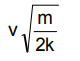Answer: (d) The maximum compression of the spring is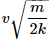Q14: Look at the drawing given in the figure which has been drawn with the ink of uniform line-thickness. The mass of ink used to draw each of the two inner circles, and each of the two-line segments is m. The mass of the ink used to draw the outer circle is 6m. The coordinates of the centres of the different parts are; outer circle (0,0), left inner circle (-a, a), right inner circle (a, a), vertical line (0, 0) and horizontal line (0, -a). The y-coordinate of the centre of mass of the ink in this drawing is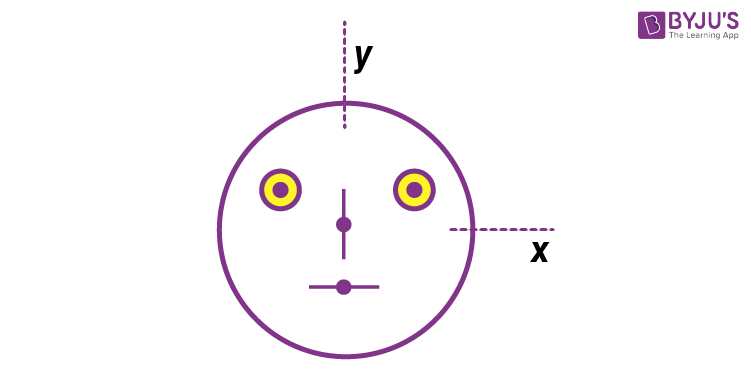(a) a/10

(b) a/8

(c) a/12

(d) a/3

Solution

Y coordinate of the centre of mass = (6m x 0 +ma+ma-ma+mx0)/(6m+m+m+m+m)

= ma/10m = a/10